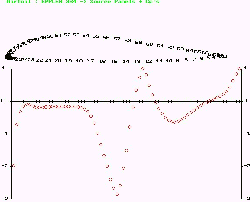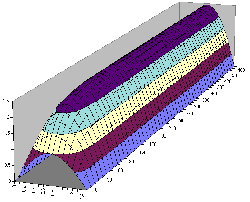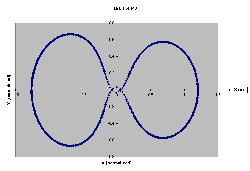Chris Good's Software Page

Welcome to my software page. I have Pascal and C code on this page in zip files for download. There is a description for each zip file and what each piece of code can be used for.

PANELS <-- click here to download

PANELS is a Pascal program that computes the pressure coefficients about any airfoil using the source panel method. It takes as input any airfoil or shape in a standard x,y format. For an example of this format, please see the airfoils contained within AIRFOIL below. It will accept any coordinate file with up to 140 x,y points. You may even have more than one airfoil in a single coordinate file. When run , the program then goes through two important steps with the user. First, it displays the airfoil(s) to the screen and asks the user to make sure that the points are in a clockwise direction. This is important as the code computes pressures to the outside of an airfoil. If the points were counter-clockwise, the pressure coefficients would be computed on the inside of the airfoil, and they would be useless. The next step displays the panels connecting all of the points and asks the user to remove any panels that are unwanted. The major reason for this capability is to remove the connecting panel between two airfoils in a single plot (such as a biplane or canard configuration). Once these two steps are complete, the program does the rest.An output text (.txt) file is created and a bitmap picture (.bmp) is also created of the final plot. Examples of these two files (with the bmp converted to jpg) are in the zip file. By taking the text output file and importing it into Excel or any other spreadsheet package, more precise plots can be made. Examples of these are in the zip file as well. The .xls files are the spreadsheets created from the output text file for a Clark-Y airfoil, a biplane configuration of the Clark-Y, a canard configuration, and a staggerwing configuration.  Here is an example of the graphic file created by PANELS.  It is a thumbnail, so click on it to see a bigger image.

If use wish to create flaps and attach them inside a coordinate file, ROTATE is another program included here that will be of help. This program takes the same type of input as above, and then allows the user to rotate that airfoil through any number of degrees clockwise or counter-clockwise. With this program, the user may create dropped flaps at any angle to see how they will change the pressure coefficients about an airfoil.

RTM.EXE is a Pascal runtime library, EGAVGA.BIN is a graphics run-time package, and BOLD.CHR is a font to be used by the program. Keep these three files in the same directory as PANELS.EXE and ROTATE.EXE.

AIRFOILS  <-- click here to download

This zip file contains 20 airfoils in the standard x,y format. They can be used by any software package that plots and modifies airfoils, such as CompuFoil. They can also be used by PANELS. For more airfoils online, please try these sites:

http://amber.aae.uiuc.edu/~m-selig/ads/coord_database.html   - Selig Airfoils

http://www.ozemail.com.au/~flyingwing/  - Flying Wing Airfoils

More software is available for airfoil plotting and modifications at these sites:

COMPCOMP <- click here to download

Composites computer is a C program that was written for a graduate composites class at UNLV. It is designed to compute the strengths in all directions of a layed-up composite. If you are familiar with composites, the S, Q, and ABD matrices will be easily recognized. The program takes either composites entered by hand and then saves them, or it will accept an input file in the form of the .lam files contained within this zip file. If you are working with composites and need these matrices and the strengths and strains that result from these matrices and loads placed upon them, this code will be of great help.

DMS <- click here to download
TOTSEC <- click here to download

These are two simple C programs.  DMS takes input at the command line and will convert latitude and longitude from degrees minutes seconds to decimal degrees, and vice versa.  TOTSEC will take any number (in seconds) and return the total number of days, hours, minutes and seconds from the beginning of the year.

TRANSFORMS <- click here to download

This is a C library of geographic coordinate transformation routines. Included with the library is a small test program to show how to use the subroutines.
EFG refers to universal, Earth center, metric (meters) coordinates
LLA refers to latitude, longitude, altitude (lat-long-alt)
AER refers to local azimuth, elevation, range (az-el-range)
XYZ refers to local standard coordinate plane
72 and 84 refers to the WGS 72 and WGS 84 Earth ellipsoids

Subroutines included are:
EFG84toLLA84 - converts from EFG in WGS 84 to Lat-Long-Alt in WGS 84
LLA84toEFG84 - converts from Lat-Long-Alt in WGS 84 to EFG in WGS 84
AERtoLLA84 - converts from local az-el-range (in meters) to Lat-Long-Alt in WGS 84
EFG84toAER - converts from EFG in WGS 84 to local az-el-range (in meters)
EFG84toEFG72 - converts from EFG in WGS 84 to EFG in WGS 72
XYZtoAER - converts from local XYZ to local az-el-range (meters in -> meters out, feet in -> feet out; consistent units)
AERtoXYZ - converts from local az-el-range to local XYZ (meters in -> meters out, feet in -> feet out; consistent units)
return_dms - accepts decimal value and returns the deg:min:sec representation

MATRIX <- click here to download

This is a C library of matrix utilities.  Included with the library is a small test program to show how to use the subroutines.

Subrountines included are:
transpose - returns the transpose of a matrix
matrix_multiply - multiplies two conformable matrices
adjoint - returns the adjoint of a square matrix
inverse - returns the inverse of a square matrix
trace - returns the trace of a square matrix
cofactor - returns the cofactor of a square matrix from position row,column
det - returns the determinate of a square matrix
minor - returns the minor of a square matrix from position row,column
print_matrix - pretty print of any size matrix
solution - this is a C version of the Pascal code "Linear" below; it will give the solution [X] of the equation [A][X]=[B] where [A] and [B] are known.  It handles tri-diagonal, sparse, and full arrays.

The following programs were written for MEG 777, High Performance Computational Science and Engineering, at UNLV.

LINEAR <- click here to download

This Pascal code can be used to solve a linear system of equations. These equations are in the form [A] [X] = [B] where [A] is a (N x N) size array and [X] & [B] are (1 x N) arrays. It has partial pivoting algorithms included in the solver, and will solve tri-diagonal, sparse, and full arrays. In its current coding it will handle up to 100 variables. RTM.EXE is a Pascal run-time library that must be in the same directory as LINEAR.EXE .  If you want a C version of this code, check out my C matrix utilities above.

CRANK <- click here to downloadThis C program is an example of the Crank-Nicolson algorithm. It is used to compute a final temperature distribution of a heat generating 1 dimensional rod. The temperature is generated by nuclear reaction. The output file was imported into Excel and a graphical representation of the temperature distribution was created. This .xls file is in the zip file.  Here is one of the images of the Excel plots created from the data.   It is a thumbnail, so click on it to see a bigger image.

RK <- click here to downloadThis C program is an example of the Runge-Kutta Time Stepping algorithm. In this case, it is used to compute the trajectory of a third body in a three body problem. The third body is the Command and Service Module on a free return orbit around the Moon. The same algorithm was used (slightly refined) by NASA for the free-return problem during the Apollo Moon Program. The output file was imported into Excel and a graphical representation of the orbit was created. This .xls file is in the zip file. Here is the image of the Excel plot created from the data.   It is a thumbnail, so click on it to see a bigger image.